Next: Removal of particle hits Up: Echelle Spectra Previous: General Description

Order Definition

The dispersion relation is defined by the following equations: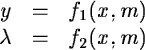(7.1)

The first of the equations 7.1 defines the position of the spectral orders, m, in the raw image, while the second equation gives, for each order, the dispersion relation in one dimension. The mapping between the spaces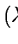and (x,y) is separated into two different equations; the first one will be discussed in this Section, while the description of the second equation will be postponed to Section 7.6.

The function f1 is approximated by a polynomial of the form(7.2)

where the coefficients aij are computed using least squares techniques on a grid (xk, yk), i.e. sample number and line number of points located within the spectral orders of the image. These points in the grid are found automatically by an order-following algorithm, normally using the FLAT or STD image.

• A first guess of the position of the orders is found on a trace perpendicular to the dispersion direction done in the middle of the flat field image, in this way we define the set of points (x0,y0m), m being the relative order number.
• For each order, the order-following algorithm finds the series of points located on the order at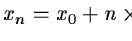for points on the right half of the order, and at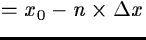for points on the left half of the order,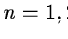integer and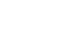is the step of the grid.

This set of points forms the basic grid with the geometric positions of the orders. Typical values of the standard deviation of the residuals of this approximation are about 0.3 to 0.1 pixel.

It is worth mentioning here that the order  following algorithm finds the center of the orders by taking the middle point with respect to the edges of the orders. The edges of the orders are detected automatically by thresholding the order profiles, perpendicular to the dispersion direction; the level of the threshold is a function of the signal in the order. The command DEFINE/ECHELLE performs the automatic order detection.

An alternative method is available, based on the Hough transform to perform the order detection and involving a tracing algorithm able to estimate an optimal threshold for each order independently. The order definition is performed as follows:

• A preprocessing of the frame is performed, including a median filtering (radx,y=2,1) to remove hot pixels and bad rows from the image. Then the background value is measured in the central area of the image and subtracted. This preprocessing assumes that the defaults are small enough to be corrected by a simple median filtering and that the interorder background is basically constant all over the image. If the above conditions are not respected, the frame must be processed by the user. The echelle command BACKGROUND/SMOOTH enables performance of a background correction at this early stage of the calibration.
• A first guess  of the position and the slope of the orders is found by processing the Hough transform of a subset of columns of the input image. The order detection by Hough transform is described in (Ballester, 1994).
• For each order, an initial threshold is estimated by measuring the pixel values in the middle of the order. The order following algorithm finds the series of points located on the order at regular steps on the grid, as describd above. The threshold is optimised in order to follow the order on the longest possible distance. If the trace of the order is lost, the algorithm extrapolates linearly the positions and attempts to skip the gap.
• For each position, the center of the order is defined as the first moment of the pixel values above the threshold:This algorithm is implemented in the command DEFINE/HOUGH. The algorithm can run in a fully automatic mode (no parameters are required apart from the name of the input frame). It is also possible to set the following parameters to enforce a given solution:
• numbers of orders to be detected.
• half-width of orders
• threshold
A practical decription of the way to use this algorithm and to optimise the parameters is described in the Appendix DNext: Removal of particle hits Up: Echelle Spectra Previous: General Description
Petra Nass
1999-06-15